output.to from Sideway
Mechanics Dynamics

Draft for Information Only

# Content

``` Mechanics: Kinetics   Geometry of Motion    Position    Distance    Displacement    Speed    Velocity    Acceleration   Newton's Laws of Motion   Inertia and Inertial Reference Frames   Idealizations```

## Mechanics: Kinetics

Kinetics is the study of the dynamic equilibrium state of an object's motion which is related to the relationship between the motion of an object and the physical properties of the object due to forces acting on it with respect to time in addition to the geometry of an object's motion.

### Geometry of Motion

#### Position

The position of an object is used to specify where the object is when describing the motion of the object. Although position is used to describe the location of an object in daily life, a position is usually considered as a vector in mechanics and physics because a position is always expressed in term of a magnitude and a direction with reference to a reference point or a zero point, that is the origin point. For example, origin O, position A, position B, and position C.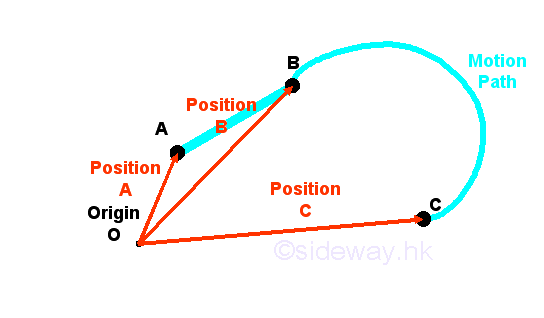#### Distance

The term distance is not a vector because a distance is only the measured magnitude between two positions with reference to each other. For example, distance between position A and position B, distance between position B and position C, and distance between position C and position A. Although distance is defined the shortest length of two positions, but travelled distance is used to described the path of the motion of the object. Therefore the total travelled distance from A to B to C is equal to the sum of path between position A and position B and path between position B and position C.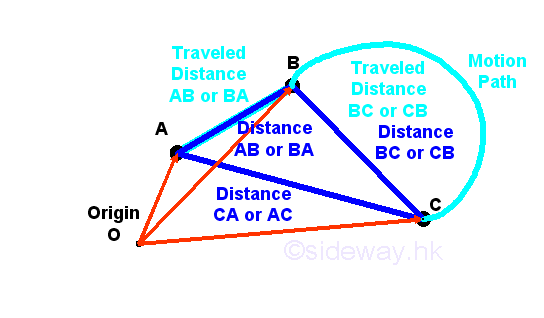#### Displacement

But, the term displacement is a vector used to specify the motion of an object, which has a magnitude and a direction also,  from one position to another position.  For example, displacement form position A to position C. Unlike the term distance, the term displacement usually refers to the shortest motion from the start position to the end position only.#### Speed

Besides where an object is or where an object is going to, how fast the object is moving is also important when describing the motion of an obeject. The generic term speed is a magnitude used to describe how fast is the object moving. Although, the term speed is usually refered to the instantaneous speed of the motion of an object, sometimes, speed is also used to describe the average speed of the motion of an object. An instantaneous speed of the motion of an object is the speed of the motion of an object at a particular instant in time or at a particular instant in position. Average speed is defined as the total travelled distance over the time interval of the time travelled. Since speed is a scalar, average speed is therefore also equal to the average of the instantaneous speed of the motion of an object within a time interval or over the total distance covered.  In other words, instantaneous speed at a particular instant in time is equal to the average speed of the motion of an object when the time travelled interval is sufficiently small such that the speed over that time interval can be considered to be a constant.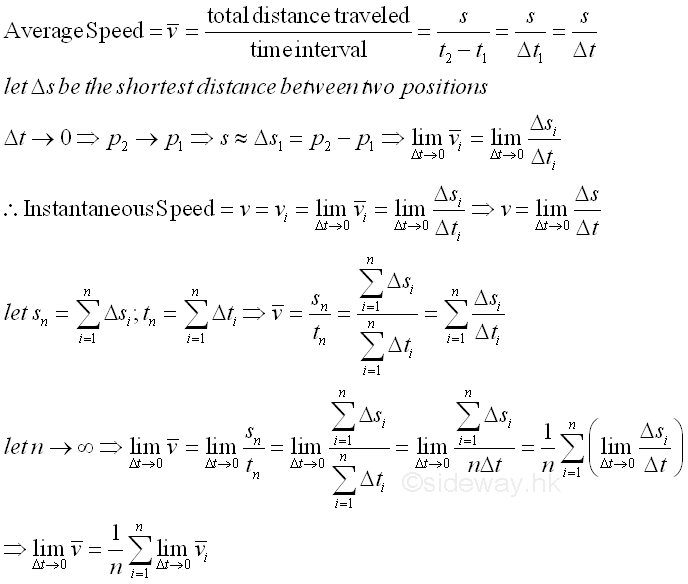#### Velocity

Although the meaning of the term velocity is often interchangeable with the term speed in daily life, however velocity and speed are different in mechanics or physics. Velocity always has both a direction and a magnitude while speed has a magnitude only. In other words, velocity is a vector and therefore unlike speed, the direction of the motion of an object should be considered when determining the velocity of the object. Similar to average speed, average velocity is defined as the displacement of an object over the time interval of the time travelled from the start position to the end position. Since velocity is a vector quantity with direction from start position to end position that is equal to end position vector minus start position vector. The magnetude of average velocity is therefore equal to the average rate of the change of position or the net displacement with respect to time. And the direction of the average velocity is same as the direction of the displacement. And therefore average velocity of an object is not always equal to the average speed of an object.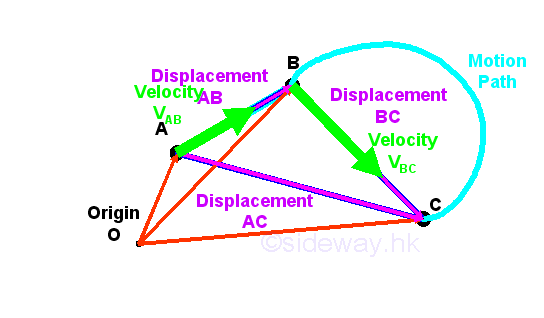Similarly, for a vector quantity, the average velocity vector is equal to the average of the vector sum of the instantaneous velocity of the motion of displacement of an object within a time interval or over the displacement covered. And the definition of instantaneous velocity is also equal to the vector representation of the rate of change of position vector with respect to time at a particular instant in time when the travelled time interval is sufficiently small such that the velocity vector over that time interval can be considered to be a constant and the travelled distance over that time interval can be considered to be equal to a linear displacement.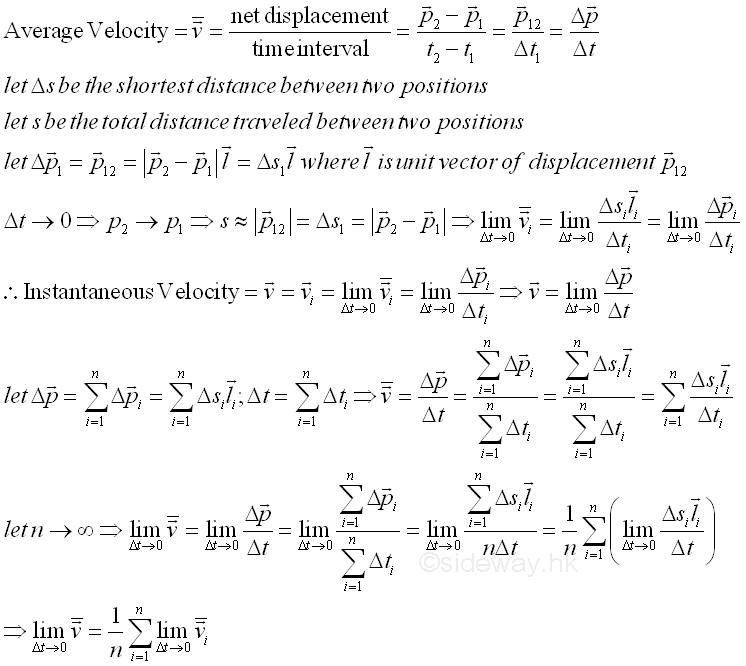#### Acceleration

In daily life, acceleration is a generic term used to describe how the speed of the motion of an object changes with time . But in mechanics or physics, acceleration is a vector quantity and is defined as the rate of an object changes its velocity from a position to another position over a time interval. In general, an object is accelerating if either the speed or velocity of the object changes with time. The acceleration of the speed of an object is a descriptive scalar quantity which is usually used to describe the movement of an object, e.g. how fast to speed up, to slow down, or to turn. While the acceleration of the velocity of an object is a vector quantity to represent the rate of net change of velocities at the start and end positions. Since acceleration is a vector quantity with direction equals to end velocity vector minus start velocity vector. The magnetude of average acceleration is therefore equal to the average rate of the change of velocity with respect to time.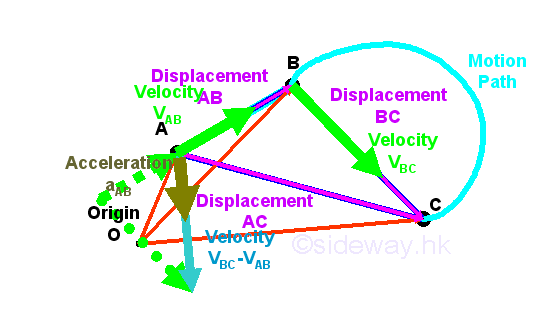Similarly, for a vector quantity, the average acceleration vector is equal to the average of the vector sum of the instantaneous acceleration of the motion of displacement of an object within a time interval or over the displacement covered. And the definition of instantaneous acceleration is also equal to the vector representation of the rate of change of velocity vector with respect to time at a particular instant in time when the travelled time interval is sufficiently small such that the acceleration vector over that time interval can be considered to be a constant, and the rate of change of the velocity over that time interval can be considered to be linear.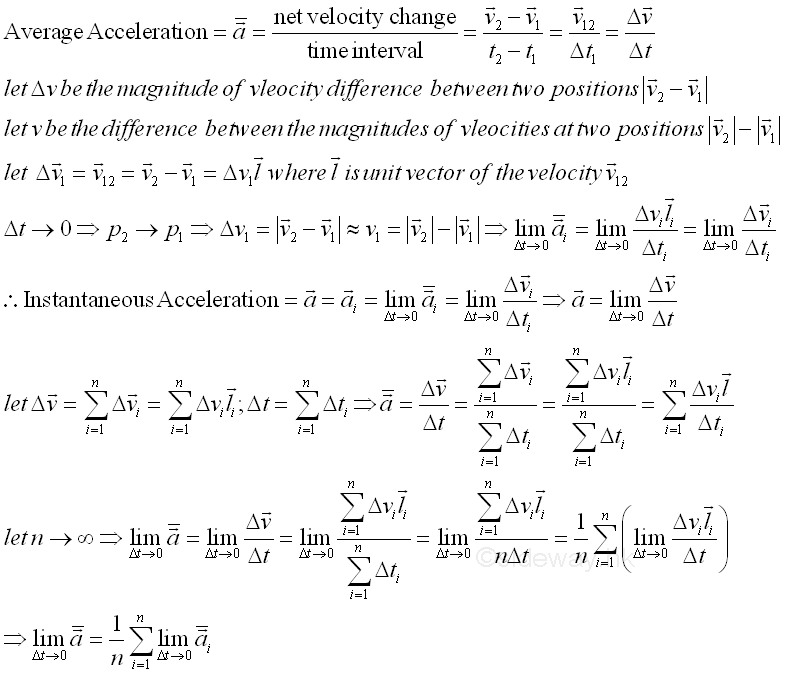### Newton's Laws of Motion

The physical properties of an object can be related to the Newton's laws of motion. According to the first law of motion, the action applied to an object to change its state of motion, either remains at rest or continues to move at a constant velocity, is described as force when frames of reference of the motion are observed in inertial frames. If the resultant action, force, acting on an object is not equal to zero, the object will accelerate with magnitude proprtional to the magnitude of the restultant action in the direction of the resultant action, force as specified by the second law of motion. From the second law, the ratio of the applied force acted upon an object and the acceleration of the oject due to the applied force is a constant value, called mass and is a characteristic of the oject.  And from the third law, there is always an equal and opposite reaction for every action. Therefore when an object exerts a force on another object, the second object will alway exerts a force of equal in magnitude but opposite in direction back to the first object along the same line of action simultaneouly.

### Inertia and Inertial Reference Frames

In static mechanics, concepts of force described in the first and third law of motion are used to tackle problems of an engineering application. The relative motion in the system is usually related by the mechanism of the system. In kinematic mechanics, concepts of relative independent motion are introduced in addition to relative dependent motion. In kinetic mechanics, the second law of motion is used to determined the motion of an object. However attention must be paid to the ccordinate system used in measuring the accelerations since an inertial frame of reference or inertial reference frame is the one in which Newton's first law of motion is valid.

Inertia of an object is defined as the tendency of an object to resist any change in its state of motion, that is to change its state from rest, to change the magnitude of its speed or to change the direction of its motion. Therefore sometime Newton's first law of motion is also referred to as the law of inertia.

A frame of reference is a standard coordinate system relative to the object which will be measured. When the law of inertial is considered, the frame, in which the law of inertia is correct, or the Newton's law of motion holds with an acceptable degree of accuracy is called an inertial frame. An inertial reference frame, also known as a Newtonian or Galilean reference frame is defined to be any uniformly moving  rigid coordinate system with time scaleframe of reference. If there is one intertial frame exists, there exists an infinite number of other inertial frames since any frame of reference moving at a constant relative velocity to the inertial frame is also an inertial frame. A non-inertial frame is an accelerating reference frame with respect to inertail frames in which the law of inertia does not hold. For example, passenger on a moving train with uniform velocity will move forward when the motion of the train is slowed down by applying brake. When taking the train as the frame of reference, the reference frame is an inertial frame when the train is moving with unifrom velocity. However the reference frame becomes an non-inertia reference frame when the train is retarding. The surface of the Earth can be considered as an inertial frame for most engineering problems because the daily rotation is quite slow.

### Idealizations

Idealizations are techniques used to simplify the application of theory in problem modeling. They arem modeling. They are

• Particle is a point mass and its size can be neglected

• Rigid Body is one single object formed by a combination of particles in which all particles remain at a fixed distance from one another and are assumed to be rigid under applied forces.

• Concentrated Force is a mathematical representation of the effect of forces acting on a rigid body by assuming a single force acts at a point on the object along a single line of force in space.

©sidewayReferences

1. I.C. Jong; B.G. rogers, 1991, Engineering Mechanics: Statics and Dynamics, Saunders College Publishing, United States of America
2. F.P. Beer; E.R. Johnston,Jr.; E.R. Eisenberg, 2004, Vector Mechanics for Engineers: Statics, McGraw-Hill Companies, Inc., New YorkID: 140900002 Last Updated: 2014/9/10 Revision: 0 Ref:Home (5)

Business

Management

HBR (3)

Information

Recreation

Hobbies (7)

Culture

Chinese (1097)

English (336)

Reference (66)

Computer

Hardware (149)

Software

Application (187)

Digitization (24)

Numeric (19)

Programming

Web (648)CSS (SC)

ASP.NET (SC)

HTML

Knowledge Base

Common Color (SC)

Html 401 Special (SC)

OS (389)

MS Windows

Windows10 (SC)

.NET Framework (SC)

DeskTop (7)

Knowledge

Mathematics

Formulas (8)

Number Theory (206)

Algebra (20)

Trigonometry (18)

Geometry (18)

Calculus (67)

Complex Analysis (21)

Engineering

Tables (8)

Mechanical

Mechanics (1)

Rigid Bodies

Statics (92)

Dynamics (37)

Fluid (5)

Control

Acoustics (19)

Biology (1)

Geography (1)

Copyright © 2000-2019 Sideway . All rights reserved Disclaimers last modified on 10 Feb 2019